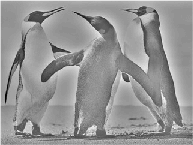MATLAB – Butterworth Highpass Filter in Image Processing

• Difficulty Level : Medium
• Last Updated : 10 May, 2020

In the field of Image Processing, Butterworth Highpass Filter (BHPF) is used for image sharpening in the frequency domain. Image Sharpening is a technique to enhance the fine details and highlight the edges in a digital image. It removes low-frequency components from an image and preserves high-frequency components.

This Butterworth highpass filter is the reverse operation of the Butterworth lowpass filter. It can be determined using the relation-where,is the transfer function of the highpass filter andis the transfer function of the corresponding lowpass filter.

The transfer function of BHPF of orderis defined as-Where,

•is a positive constant. BHPF passes all the frequencies greater thanvalue without attenuation and cuts off all the frequencies less than it.
• Thisis the transition point between H(u, v) = 1 and H(u, v) = 0, so this is termed as cutoff frequency. But instead of making a sharp cut-off (like, Ideal Highpass Filter (IHPF)), it introduces a smooth transition from 0 to 1 to reduce ringing artifacts.
•is the Euclidean Distance from any point (u, v) to the origin of the frequency plane, i.e,Approach:
Step 1: Input – Read an image
Step 2: Saving the size of the input image in pixels
Step 3: Get the Fourier Transform of the input_image
Step 4: Assign the orderand cut-off frequencyStep 5: Designing filter: Butterworth High Pass Filter
Step 6: Convolution between the Fourier Transformed input image and the filtering mask
Step 7: Take Inverse Fourier Transform of the convoluted image
Step 8: Display the resultant image as output

Implementation in MATLAB:

 % MATLAB Code | Butterworth High Pass Filter      % Reading input image : input_imageinput_image = imread('[name of input image file].[file format]');  % Saving the size of the input_image in pixels-% M : no of rows (height of the image)% N : no of columns (width of the image)[M, N] = size(input_image);  % Getting Fourier Transform of the input_image% using MATLAB library function fft2 (2D fast fourier transform)FT_img = fft2(double(input_image));  % Assign the order valuen = 2; % one can change this value accordingly  % Assign Cut-off FrequencyD0 = 10; % one can change this value accordingly  % Designing filteru = 0:(M-1);v = 0:(N-1);idx = find(u > M/2);u(idx) = u(idx) - M;idy = find(v > N/2);v(idy) = v(idy) - N;  % MATLAB library function meshgrid(v, u) returns % 2D grid which contains the coordinates of vectors % v and u. Matrix V with each row is a copy of v % and matrix U with each column is a copy of u [V, U] = meshgrid(v, u);  % Calculating Euclidean DistanceD = sqrt(U.^2 + V.^2);  % determining the filtering maskH = 1./(1 + (D0./D).^(2*n));  % Convolution between the Fourier Transformed % image and the maskG = H.*FT_img;  % Getting the resultant image by Inverse Fourier Transform % of the convoluted image using MATLAB library function  % ifft2 (2D inverse fast fourier transform)   output_image = real(ifft2(double(G)));     % Displaying Input Image and Output Image subplot(2, 1, 1), imshow(input_image), subplot(2, 1, 2), imshow(output_image, [ ]);

Input Image –Output:Note: The result of BHPF is much smoother than IHPF. Here, the boundaries are much less distorted, even for the smallest value of cutoff frequency. The transition into higher values of cutoff frequencies is much smoother with the BHPF.

My Personal Notes arrow_drop_up